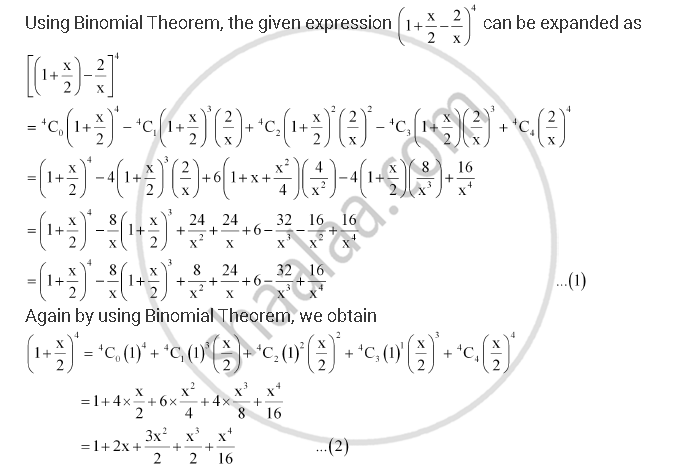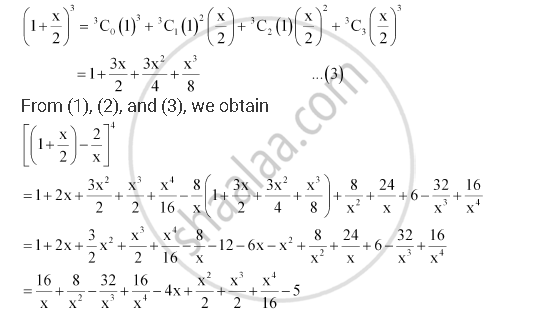CBSE (Arts) Class 11CBSE
Share
Notifications

View all notifications
Books Shortlist
Your shortlist is empty

# Expand Using Binomial Theorem (1+ X/2 - 2/X)^4, X != 0 - CBSE (Arts) Class 11 - Mathematics

Login
Create free account

Forgot password?
ConceptBinomial Theorem for Positive Integral Indices

#### Question

Expand using Binomial Theorem (1+ x/2 - 2/x)^4, x != 0

#### SolutionIs there an error in this question or solution?

#### APPEARS IN

Solution Expand Using Binomial Theorem (1+ X/2 - 2/X)^4, X != 0 Concept: Binomial Theorem for Positive Integral Indices.
S